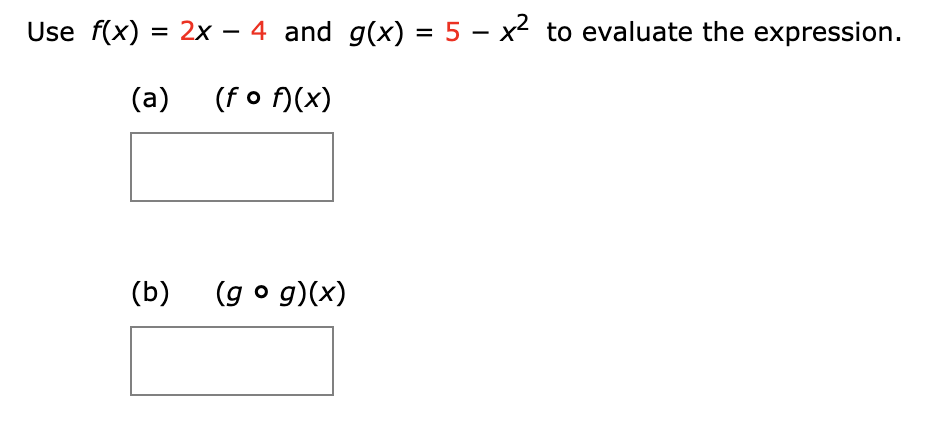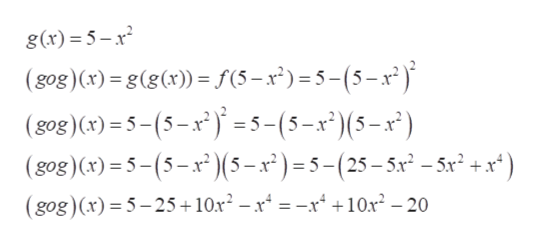# Use f(x) 2x - 4 and g(x) = 5 - x2 to evaluate the expression(a)(fo (x)(b)(g o g)(x)

Question
12 viewshelp_outlineImage TranscriptioncloseUse f(x) 2x - 4 and g(x) = 5 - x2 to evaluate the expression (a) (fo (x) (b) (g o g)(x) fullscreen
check_circle

Step 1

Given f(x) and g(x)

Step 2

(fof)(x)= f(f(x)). Substitute f(x) in f(x) itself

Step 3

(gog)(x)= g(g(x)). Substitut...help_outlineImage Transcriptioncloseg(x) = 5-1 (gog)(x) = g(g(x)) = f(5-x)=5-(5-x2) (gog)(x)=5-(5-x)=5-(5-x")(5-x) (gog)(x)-5-(5-x(5-x')=5-(25-5x -5x +x*) (gog)(x) 5-25+ 10x2 - x4 = -r4 +10x2 -20 fullscreen

### Want to see the full answer?

See Solution

#### Want to see this answer and more?

Solutions are written by subject experts who are available 24/7. Questions are typically answered within 1 hour.*

See Solution
*Response times may vary by subject and question.
Tagged in

### Functions